Get inspired by the success stories of our students in IIT JAM MS, ISI  MStat, CMI MSc DS.  Learn More

# ISI MStat PSB 2006 Problem 8 | Bernoullian BeautyThis is a very beautiful sample problem from ISI MStat PSB 2006 Problem 8. It is based on basic idea of Maximum Likelihood Estimators, but with a bit of thinking. Give it a thought !

## Problem- ISI MStat PSB 2006 Problem 8

Let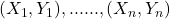be a random sample from the discrete distributions with joint probability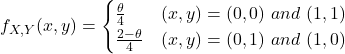with. Find the maximum likelihood estimator of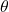.

### Prerequisites

Maximum Likelihood Estimators

Indicator Random Variables

Bernoulli Trials

## Solution :

This is a very beautiful Problem, not very difficult, but her beauty is hidden in her simplicity, lets explore !!

Observe, that the given pmf is as good as useless while taking us anywhere, so we should think out of the box, but before going out of the box, lets collect whats in the box !

So, from the given pmf we get,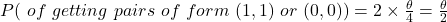,

Similarly,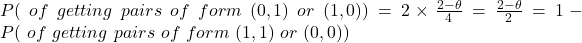So, clearly it is giving us a push towards involving Bernoulli trials, isn't it !!

So, lets treat the pairs with match, .i.e.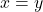, be our success, and the other possibilities be failure, then our success probability is, where. So, if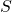be the number of successful pairs in our given sample of size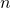, then it is evident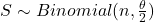.

So, now its simplified by all means, and we know the MLE of population proportion in binomial is the proportion of success in the sample,

Hence,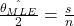, where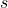is the number of those pairs in our sample where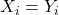.

So,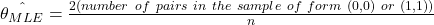.

Hence, we are done !!

## Food For Thought

Say,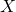and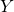are two independent exponential random variable with means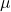andrespectively. But you observe two other variables,and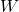, such that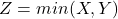andtakes the valuewhen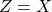and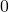otherwise. Can you find the MLEs of the parameters ?

Give it a try !!

## Subscribe to Cheenta at Youtube

This is a very beautiful sample problem from ISI MStat PSB 2006 Problem 8. It is based on basic idea of Maximum Likelihood Estimators, but with a bit of thinking. Give it a thought !

## Problem- ISI MStat PSB 2006 Problem 8

Letbe a random sample from the discrete distributions with joint probabilitywith. Find the maximum likelihood estimator of.

### Prerequisites

Maximum Likelihood Estimators

Indicator Random Variables

Bernoulli Trials

## Solution :

This is a very beautiful Problem, not very difficult, but her beauty is hidden in her simplicity, lets explore !!

Observe, that the given pmf is as good as useless while taking us anywhere, so we should think out of the box, but before going out of the box, lets collect whats in the box !

So, from the given pmf we get,,

Similarly,So, clearly it is giving us a push towards involving Bernoulli trials, isn't it !!

So, lets treat the pairs with match, .i.e., be our success, and the other possibilities be failure, then our success probability is, where. So, ifbe the number of successful pairs in our given sample of size, then it is evident.

So, now its simplified by all means, and we know the MLE of population proportion in binomial is the proportion of success in the sample,

Hence,, whereis the number of those pairs in our sample where.

So,.

Hence, we are done !!

## Food For Thought

Say,andare two independent exponential random variable with meansandrespectively. But you observe two other variables,and, such thatandtakes the valuewhenandotherwise. Can you find the MLEs of the parameters ?

Give it a try !!

## Subscribe to Cheenta at Youtube

This site uses Akismet to reduce spam. Learn how your comment data is processed.

### Knowledge Partner